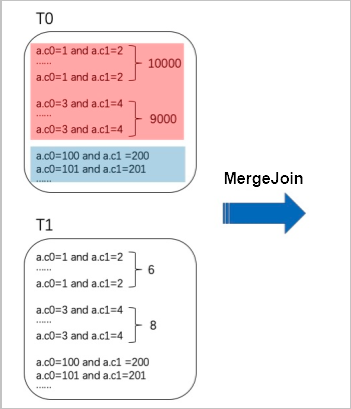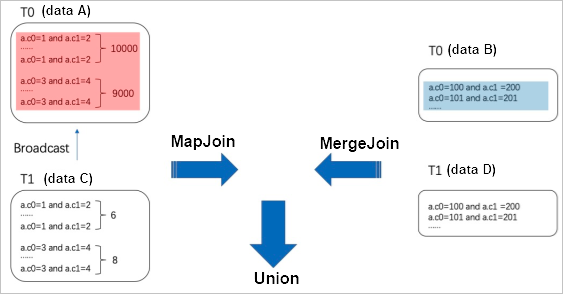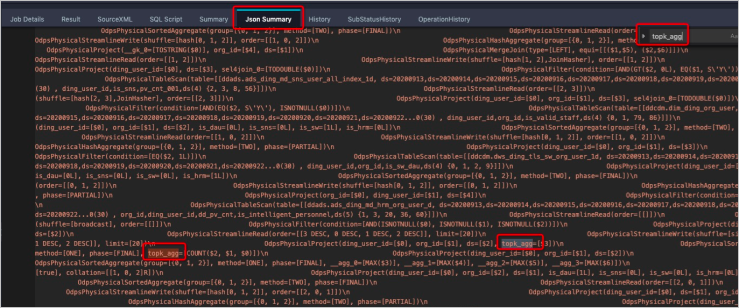If two tables you want to join have hot key values, a long tail may occur. In this case, you can use SKEWJOIN HINT to automatically or manually extract hot key values from the two tables, separately calculate the join result of hot key values and the join result of non-hot key values, and then join the calculated data. This accelerates the JOIN operation.

## Usage

MAP JOIN can be performed only after you add the SKEWJOIN HINT ```/*+ skewJoin(<table_name>[(<column1_name>[,<column2_name>,...])][((<value11>,<value12>)[,(<value21>,<value22>)...])] */``` to the SELECT statement. In this hint, table_name indicates the name of a skewed table, column_name indicates the name of a skewed column, and value indicates a skewed key value.

``````-- Method 1: Include the alias of the table in SKEWJOIN HINT.
select /*+ skewjoin(a) */ * from T0 a join T1 b on a.c0 = b.c0 and a.c1 = b.c1;
-- Method 2: Include the table name and possibly skewed columns in SKEWJOIN HINT. In the following statement, the c0 and c1 columns of table a are skewed columns.
select /*+ skewjoin(a(c0, c1)) */ * from T0 a join T1 b on a.c0 = b.c0 and a.c1 = b.c1 and a.c2 = b.c2;
-- Method 3: Include the table name, columns, and skewed hot key values in SKEWJOIN HINT. If skewed key values are of the STRING type, enclose each value with double quotation marks. In the following statement, (a.c0=1 and a.c1="2") and (a.c0=3 and a.c1="4") contain skewed hot key values.
select /*+ skewjoin(a(c0, c1)((1, "2"), (3, "4"))) */ * from T0 a join T1 b on a.c0 = b.c0 and a.c1 = b.c1 and a.c2 = b.c2;``````
Note Method 3 is more efficient than Method 1 and Method 2.

## Implementation

Hot key values are the key values that appear multiple times in a table. In the following figure, the red part has 10,000 records of `a.c0=1 and a.c1=2` and 9,000 records of `a.c0=3 and a.c1 = 4`.If SKEWJOIN HINT is not used and the T0 and T1 tables contain large amounts of data, only the MERGE JOIN statement can be executed on the two tables. In this case, the same hot key values are shuffled to a single node. As a result, data skew occurs. After SKEWJOIN HINT is used, the optimizer runs an Aggregate to dynamically obtain top 20 hot key values based on the number of their records. The optimizer separately extracts a hot key value (data A) and a non-hot key value (data B) from the T0 table, and separately extracts a value (data C) that can be joined with data A and a value (data D) that cannot be joined with data A from the T1 table. Then, the MAP JOIN statement is executed on data A and data C (with a small data volume) and the MERGE JOIN statement is executed on data B and data D. The UNION statement is executed on the results of the MAP JOIN and MERGE JOIN statements to generate the final result, as shown in the following figure.## Precautions

• When you use SKEWJOIN HINT for JOIN statements, take note of the following limits:
• INNER JOIN: SKEWJOIN HINT can be specified for the left or right table in the INNER JOIN statement.
• LEFT JOIN, SEMI JOIN, and ANTI JOIN: SKEWJOIN HINT can be specified only for the left table.
• RIGHT JOIN: SKEWJOIN HINT can be specified only for the right table.
• FULL JOIN: SKEWJOIN HINT is not supported.
• We recommend that you add SKEWJOIN HINT only to the JOIN statements that will cause data skew. This is because an Aggregate is run after SKEWJOIN HINT is added, which slows down the JOIN operation.
• The data type of Left Side Join Key must be the same as that of Right Side Join Key for the JOIN statement to which SKEWJOIN HINT is added. If the data types are different, SKEWJOIN HINT becomes ineffective. In the preceding example, the data types of a.c0 and b.c0 must be the same and the data types of a.c1 and b.c1 must be the same. To ensure data type consistency, you can use the CAST function to convert the join keys in subqueries. Examples:
``````create table T0(c0 int, c1 int, c2 int, c3 int);
create table T1(c0 string, c1 int, c2 int);
-- Method 1:
select /*+ skewjoin(a) */ * from T0 a join T1 b on cast(a.c0 as string) = cast(b.c0 as string) and a.c1 = b.c1;
-- Method 2:
select /*+ skewjoin(b) */ * from (select cast(a.c0 as string) as c00 from T0 a) b join T1 c on b.c00 = c.c0;``````
• After SKEWJOIN HINT is added, the optimizer runs an Aggregate to obtain top 20 hot key values, by default. You can run the `set odps.optimizer.skew.join.topk.num = xx;` command to specify the number of hot key values that the optimizer can obtain.
• SKEWJOIN HINT allows you to add a hint only for the left or right table involved in the JOIN statement.
• In the JOIN statement to which SKEWJOIN HINT is added, `left key = right key` must be included. SKEWJOIN HINT cannot be added to the CARTESIAN JOIN statement.
• The following statement shows how to use SKEWJOIN HINT with other hints. Note that SKEWJOIN HINT cannot be added to the JOIN statement to which MAPJOIN HINT is added.
``select /*+ mapjoin(c), skewjoin(a) */ * from T0 a join T1 b on a.c0 = b.c3 join T2 c on a.c0 = c.c7;``
• On the Json Summary tab of Logview, you can search for the topk_agg field. If such a field exists, as shown in the following figure, SKEWJOIN HINT has taken effect.